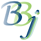# SQL — Aggregate Functions

GROUP BY
works in conjunction with SQL aggregate functions (AVG(), SUM(), COUNT(), MIN(), and MAX(). These functions perform operations for each record in an individual group or produce a summary value for each set.

## Aggregate Function Syntax

The following identifies the general aggregate function syntax:

AGG(<DISTINCT | ALL (default)> colname)

 Parameter Description AGG Name of an aggregate function colname Column from a table. If DISTINCT is specified, then only distinct colname values will be considered for aggregation. If ALL is specified, then all values will be considered for aggregation.

The following identifies the syntax for the (AVG(), SUM(), COUNT(), MIN(), and MAX() aggregate functions:

 Function Syntax Returns SUM() SUM( colname Summation of all colname numeric values for each group. MIN() MIN( colname) Lowest colname numeric or string values for each group. MAX() MAX( colname) Highest colname numeric or string values for each group. AVG() AVG( colname) Arithmetic mean for numeric colname values for each group. COUNT() COUNT(*) Number of records in each group. COUNT(colname) Count of distinct colname items in each group.

## Example

In the following example, the average SALES_LY will be determined from the entire CUSTOMER table. Since GROUP BY is not specified, the result set is only be a single row:

select AVG(sales_ly) from customer

In the following example, the average sales grouped by salesperson, and a row is shown for each salesperson:

select AVG(sales_ly), salesperson from customer group by salesperson